• 累计撰写 113 篇文章
• 累计收到 306 条评论

# 最简单入门深度学习2020-10-24 / 0 评论 / 1,062 阅读 / 正在检测是否收录...

# 最简单入门深度学习

• 概述
• 线性模型：单神经元
• 非线性模型：深度神经网络
• 模型训练：随机梯度下降
• 验证模型：过拟合和欠拟合
• 提升性能：Dropout和Batch Normalization
• 分类问题

## 单个神经元

### 线性单元

y = w*x+b

`x`为输入，神经元连接的权重为`w``w`的更新就是神经网络学习的过程，`b`为偏差，它与输入没有关系，偏差允许神经元不依赖输入来修改输出，`y`是神经元的输出，即公式`y=w*x+b`的结果；

### 多个输入

y = w_0x_0 + w_1x_1 + w_2*x_2 + b

### Keras中使用线性单元

``````from tensorflow import keras
from tensorflow.keras import layers

# Create a network with 1 linear unit
model = keras.Sequential([
layers.Dense(units=1, input_shape=)
])
``````

## 深度神经网络

### 激活函数

y=w*x+b

y = max(0, w*x+b)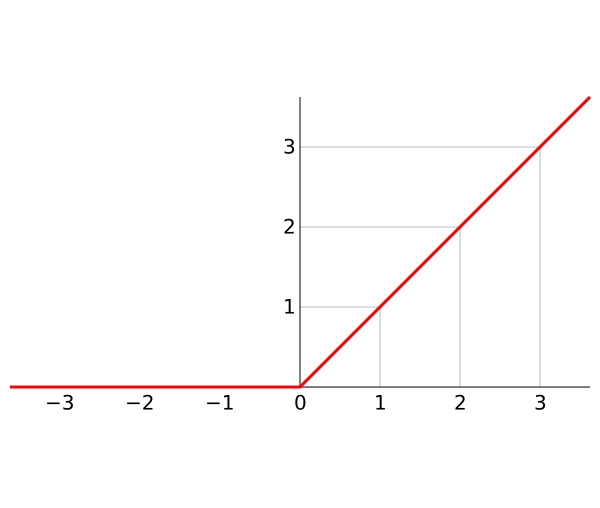### 堆叠dense层

``````from tensorflow.keras import layers

model = keras.Sequential([
# the hidden ReLU layers
layers.Dense(units=4, activation='relu', input_shape=),
layers.Dense(units=3, activation='relu'),
# the linear output layer
layers.Dense(units=1),
])
``````

### 深度神经网络练习

relu：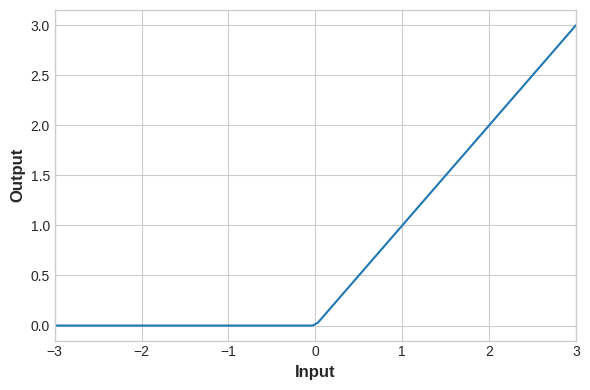elu：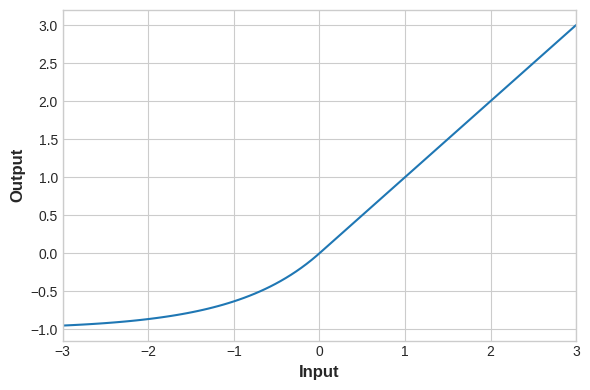selu：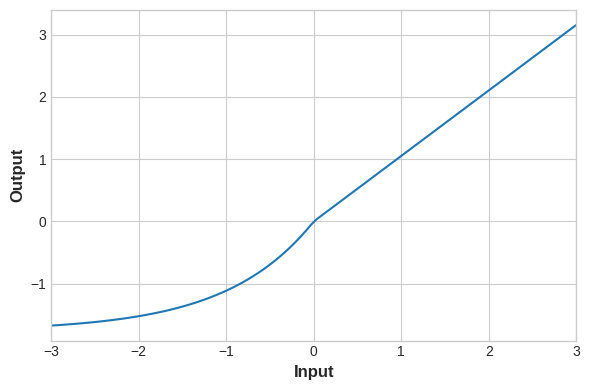swish：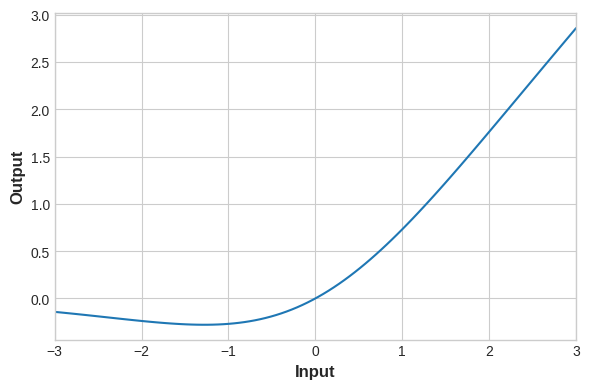## 随机梯度下降

• 损失函数：衡量模型预测结果好坏；
• 优化方法：指导模型如何去修改权重；

### 优化方法 - 随机梯度下降

1. 抽样部分训练数据，通过模型运行得到预测结果`y_pred`
2. 测量这些`y_pred``y_true`之间的损失函数值；
3. 通过损失更小的方向来修改权重；

#### 添加损失函数和优化方法

``````model.compile(
loss="mae",
)
``````

### 例子 - 红酒品质

fixed acidity volatile acidity citric acid residual sugar chlorides free sulfur dioxide total sulfur dioxide density pH sulphates alcohol quality
10.8 0.470 0.43 2.10 0.171 27.0 66.0 0.99820 3.17 0.76 10.8 6
8.1 0.820 0.00 4.10 0.095 5.0 14.0 0.99854 3.36 0.53 9.6 5
9.1 0.290 0.33 2.05 0.063 13.0 27.0 0.99516 3.26 0.84 11.7 7
10.2 0.645 0.36 1.80 0.053 5.0 14.0 0.99820 3.17 0.42 10.0 6

``````from tensorflow import keras
from tensorflow.keras import layers

model = keras.Sequential([
layers.Dense(512, activation='relu', input_shape=),
layers.Dense(512, activation='relu'),
layers.Dense(512, activation='relu'),
layers.Dense(1),
])
``````

``````model.compile(
loss='mae',
)
``````

``````history = model.fit(
X_train, y_train,
validation_data=(X_valid, y_valid),
batch_size=256,
epochs=10,
)
``````

``````import pandas as pd

# convert the training history to a dataframe
history_df = pd.DataFrame(history.history)
# use Pandas native plot method
history_df['loss'].plot();
``````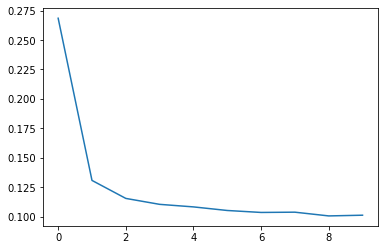### 模型训练练习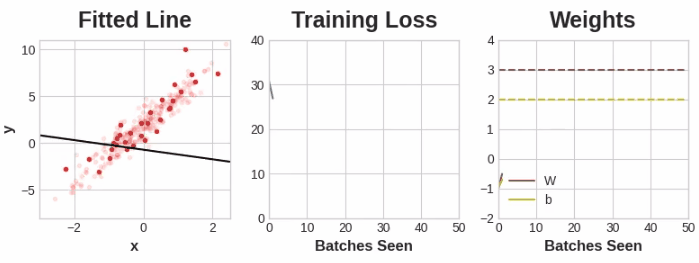## 过拟合和欠拟合

### 容量

``````假设当前网络有两层，每一层都有3个神经元，则其组合为3*3=9，此时我们要增加2个神经元：

``````

### 提前停止训练

#### 增加Early Stopping

`keras`通过callback的方式添加Early Stopping，所谓callback指的是在每次epoch后运行的内容，用于判断是否应该终止训练过程：

``````from tensorflow.keras.callbacks import EarlyStopping

early_stopping = EarlyStopping(
min_delta=0.001, # minimium amount of change to count as an improvement
patience=20, # how many epochs to wait before stopping
restore_best_weights=True,
)
``````

### 例子 - 使用Early Stopping训练模型

fixed acidity volatile acidity citric acid residual sugar chlorides free sulfur dioxide total sulfur dioxide density pH sulphates alcohol quality
10.8 0.470 0.43 2.10 0.171 27.0 66.0 0.99820 3.17 0.76 10.8 6
8.1 0.820 0.00 4.10 0.095 5.0 14.0 0.99854 3.36 0.53 9.6 5
9.1 0.290 0.33 2.05 0.063 13.0 27.0 0.99516 3.26 0.84 11.7 7
10.2 0.645 0.36 1.80 0.053 5.0 14.0 0.99820 3.17 0.42 10.0 6

``````from tensorflow import keras
from tensorflow.keras import layers
from tensorflow.keras.callbacks import EarlyStopping

early_stopping = EarlyStopping(
min_delta=0.001, # minimium amount of change to count as an improvement
patience=20, # how many epochs to wait before stopping
restore_best_weights=True,
)

model = keras.Sequential([
layers.Dense(512, activation='relu', input_shape=),
layers.Dense(512, activation='relu'),
layers.Dense(512, activation='relu'),
layers.Dense(1),
])
model.compile(
loss='mae',
)

history = model.fit(
X_train, y_train,
validation_data=(X_valid, y_valid),
batch_size=256,
epochs=500,
callbacks=[early_stopping],
verbose=0,  # turn off training log
)

history_df = pd.DataFrame(history.history)
history_df.loc[:, ['loss', 'val_loss']].plot();
print("Minimum validation loss: {}".format(history_df['val_loss'].min()))
``````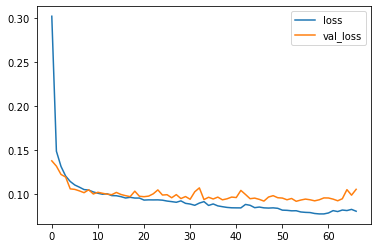### 过拟合和欠拟合的练习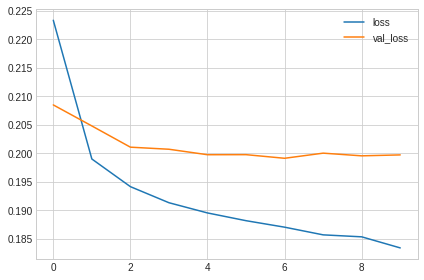## Dropout和Batch Normalization

### Dropout

Dropout层有助于纠正过拟合问题，在每次训练迭代中，随机的去掉网络层中的一部分输入单元，使得模型难以从训练数据学习到错误的模式，取而代之的是模型会搜索更普遍适用的模式，也就是具有更好鲁棒性的模式，借此解决过拟合问题；

#### 增加Dropout

`keras`中，Drouput作为层使用，作用于其下的一层，通过参数`rate`指定随机取出的比例：

``````keras.Sequential([
# ...
layer.Dropout(rate=0.3), # apply 30% dropout to the next layer
layer.Dense(16),
# ...
])
``````

### Batch Normalization

Batch Normalization类似SKLearn里的StandardScaler和MinMaxScaler的作用，用于将输入特征的量纲统一，避免因为量纲不同导致对于预测结果影响的权重差异；

#### 增加Batch Normalization

``````layers.Dense(16, activation='relu'),
layers.BatchNormalization(),
``````

``````layers.Dense(16),
layers.BatchNormalization(),
layers.Activation('relu'),
``````

### 例子 - 使用Dropout和Batch Normalization

``````from tensorflow import keras
from tensorflow.keras import layers

model = keras.Sequential([
layers.Dense(1024, activation='relu', input_shape=),
layers.Dropout(0.3),
layers.BatchNormalization(),
layers.Dense(1024, activation='relu'),
layers.Dropout(0.3),
layers.BatchNormalization(),
layers.Dense(1024, activation='relu'),
layers.Dropout(0.3),
layers.BatchNormalization(),
layers.Dense(1),
])
``````

``````model.compile(
loss='mae',
)

history = model.fit(
X_train, y_train,
validation_data=(X_valid, y_valid),
batch_size=256,
epochs=100,
verbose=0,
)

# Show the learning curves
history_df = pd.DataFrame(history.history)
history_df.loc[:, ['loss', 'val_loss']].plot();
``````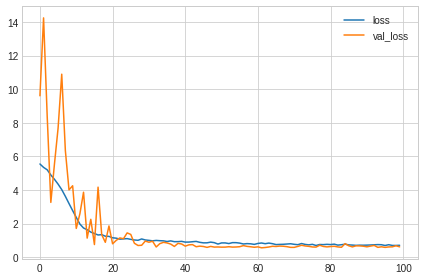### Dropout和Batch Normalization练习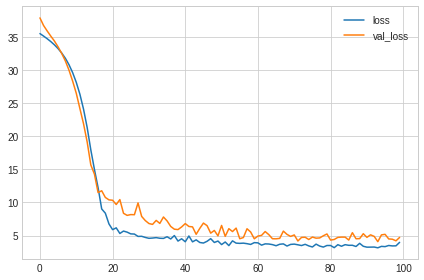## 分类问题

• 损失函数：分类与回归在损失函数应用上不同，比如MAE和准确率；
• 输出层输出类型：也就是网络结构最后一层输出的内容，之前都是数值，如果是二分类问题，则应该是0/1；

### Sigmoid函数

Sigmoid函数同样作为激活函数，它可以将实数输出映射到0到1之间，也就是通常的概率范围，而不管是准确率还是交叉熵等都可以利用概率来计算得到；

Sigmoid函数图像如下，上一个使用它的地方是逻辑回归，同样是将线性回归的结果映射到0和1之间：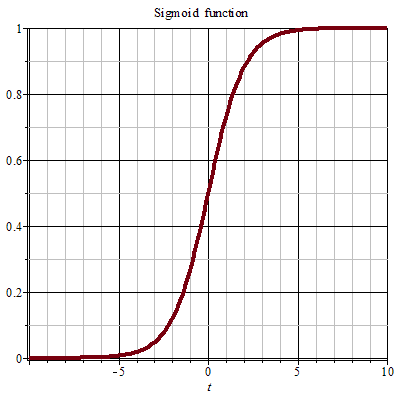### 例子 - 二分类

V1 V2 V3 V4 V5 V6 V7 V8 V9 V10 ... V26 V27 V28 V29 V30 V31 V32 V33 V34 Class
1 0 0.99539 -0.05889 0.85243 0.02306 0.83398 -0.37708 1.00000 0.03760 ... -0.51171 0.41078 -0.46168 0.21266 -0.34090 0.42267 -0.54487 0.18641 -0.45300 good
1 0 1.00000 -0.18829 0.93035 -0.36156 -0.10868 -0.93597 1.00000 -0.04549 ... -0.26569 -0.20468 -0.18401 -0.19040 -0.11593 -0.16626 -0.06288 -0.13738 -0.02447 bad
1 0 1.00000 -0.03365 1.00000 0.00485 1.00000 -0.12062 0.88965 0.01198 ... -0.40220 0.58984 -0.22145 0.43100 -0.17365 0.60436 -0.24180 0.56045 -0.38238 good
1 0 1.00000 -0.45161 1.00000 1.00000 0.71216 -1.00000 0.00000 0.00000 ... 0.90695 0.51613 1.00000 1.00000 -0.20099 0.25682 1.00000 -0.32382 1.00000 bad
1 0 1.00000 -0.02401 0.94140 0.06531 0.92106 -0.23255 0.77152 -0.16399 ... -0.65158 0.13290 -0.53206 0.02431 -0.62197 -0.05707 -0.59573 -0.04608 -0.65697 good

``````from tensorflow import keras
from tensorflow.keras import layers

model = keras.Sequential([
layers.Dense(4, activation='relu', input_shape=),
layers.Dense(4, activation='relu'),
layers.Dense(1, activation='sigmoid'),
])
``````

``````model.compile(
loss='binary_crossentropy',
metrics=['binary_accuracy'],
)
``````

``````early_stopping = keras.callbacks.EarlyStopping(
patience=10,
min_delta=0.001,
restore_best_weights=True,
)

history = model.fit(
X_train, y_train,
validation_data=(X_valid, y_valid),
batch_size=512,
epochs=1000,
callbacks=[early_stopping],
verbose=0, # hide the output because we have so many epochs
)
``````

``````history_df = pd.DataFrame(history.history)
# Start the plot at epoch 5
history_df.loc[5:, ['loss', 'val_loss']].plot()
history_df.loc[5:, ['binary_accuracy', 'val_binary_accuracy']].plot()

print(("Best Validation Loss: {:0.4f}" +\
"\nBest Validation Accuracy: {:0.4f}")\
.format(history_df['val_loss'].min(),
history_df['val_binary_accuracy'].max()))
``````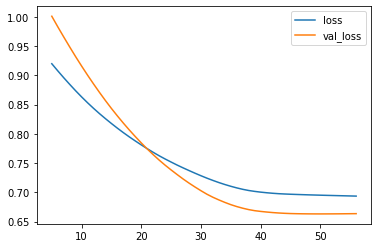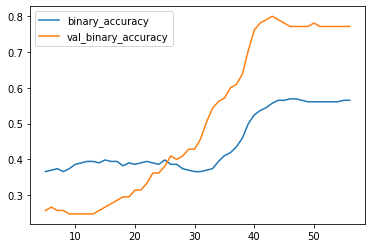### 分类练习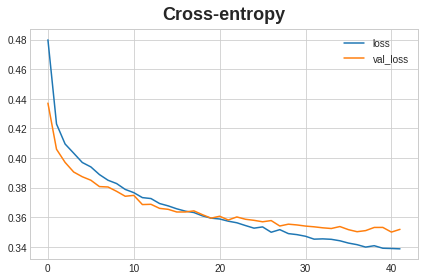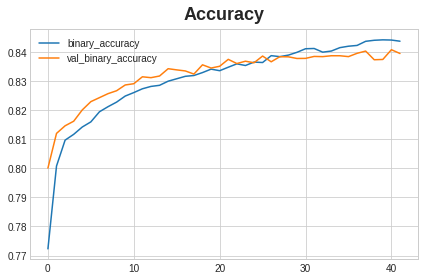## 最后的最后0